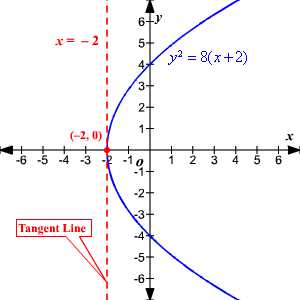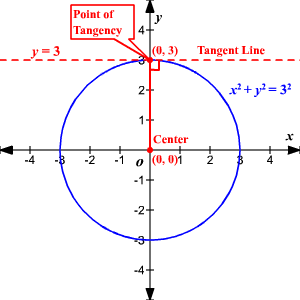# Tangent Line

A tangent line is a line that touches a curve in exactly one point.  More formally, it is a differentiable curve at a point where the slope of the curve equals the slope of a line.A tangent line to a circle is perpendicular to the radius drawn to the point of tangency.